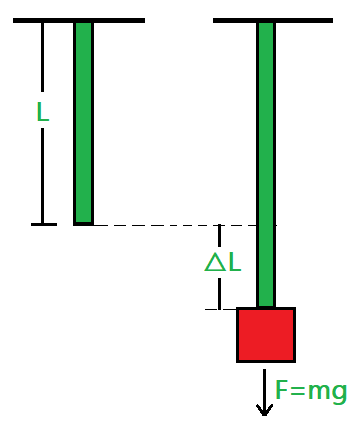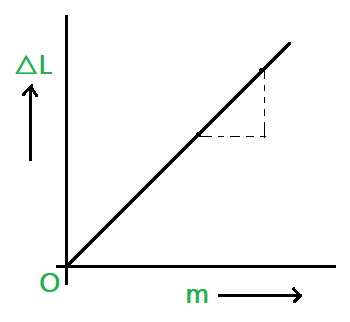# Young’s Modulus

• Last Updated : 02 Jun, 2021

The Young’s modulus is named after Thomas Young, a British physicist. The relationship between stress (force per unit area) and strain (proportional deformation in an object) is defined by Young’s modulus.  When a certain load is added to a rigid material, it deforms. When the weight is withdrawn from an elastic material, the body returns to its original form. Beyond a minimal degree of deformation, often materials are not linear and elastic. Only linear elastic surfaces have a steady Young’s modulus.

Like the Bulk modulus, Poisson’s ratio, and shear modulus, Young’s modulus is also an elastic constant. Therefore, before understanding the concept of Young’s modulus let’s first learn about the elastic constants.

### Elastic Constants

The elastic constants are those constants that determine the deformation produced by a given stress system acting on the material. There are many types of elastic constants, like Bulk modulus, Young’s modulus, or modulus of Elasticity, Poisson’s Ratio, and Shear modulus, or modulus of rigidity. As a deforming force acts on an object, it causes the solid’s original dimension to shift. In such situations, the relationship between elastic constants can be used to determine the degree of deformation.

Following are the main elastic constants that need to be learned:

1.  Bulk Modulus: It is given by the ratio of pressure (P) applied to the corresponding relative decrease in the volume or volumetric strain (∈V) of the material. Mathematically, it is defined as:

K = P / ∈V = P / -(∆V/V0)

where ∆V is the change of the volume of the material due to the compression and V0 is the Initial volume of the material.

The value is denoted with a symbol of K, and it has the dimension of force per unit area.

It is expressed in the units per square inch (psi) in the English system and Newtons per square meter (N/m2) in the metric system.

2.  Young’s Modulus: This is a measure of a solid’s stiffness or resistance to elastic deformation under load. It relates stress (force per unit area) to strain (proportional deformation) along an axis or line. Mathematically, it is defined as:

Y = (F/A) / -(∆L/L0)

where ∆L is the change of the length of the material due to the compression and L0 is the initial length of the material.

The value is denoted with a symbol of Y, and it has the dimension of force per unit area.

The SI unit for Young’s modulus is Pascal (Pa), values are most often expressed in terms of megapascal (MPa) or Newtons per square millimeter (N/mm2).

3.  Shear Modulus or Modulus of Rigidity: The ratio of shearing stress (or) tangential stress and shearing strain (or) tangential strain is called modulus of rigidity. Mathematically, it is defined as:

η = (F/A) / ∅

where F is the force applied on a unit surface area of the body A and ∅ is the shearing strain.

The value is denoted with a symbol of η.

It is expressed Pascals (Pa) in the SI system.

Now, Lets discuss the topic Young’s modulus of elasticity further in detail as:

### Young’s Modulus of Elasticity

Young’s modulus is a mathematical constant. It was named after Thomas Young, an 18th-century English physician and scientist. It defines the elastic characteristics of a solid that is subjected to tension or compression only in one direction. As an example, consider a metal rod that returns to its original length after being stretched or squeezed longitudinally.

It is a measurement of a material’s capacity to endure changes in length when subjected to longitudinal tension or compression. It’s also known as the modulus of elasticity. We compute it by dividing It is calculated as the longitudinal stress divided by the strain. In the instance of a tensioned metal bar, both stress and strain may be stated.

Young’s Modulus, also known as Elastic Modulus or Tensile Modulus, is a mechanical property measurement of linear elastic solids such as rods, wires, and so on. Other numbers exist that give us with a measure of a material’s elastic characteristics. Bulk modulus and shear modulus are two examples. However, the value of Young’s Modulus is most commonly utilised. This is because it provides information about a material’s tensile elasticity.

When a material is compressed or stretched, it experiences elastic deformation and returns to its original shape when the load is released. When a flexible material deforms, it deforms more than when a rigid substance deforms. In other words it can be interpreted as:

• A solid with a low Young’s modulus value is elastic.
• A solid with a high Young’s modulus value is inelastic or stiff.

Young’s modulus is described as a material’s mechanical ability to tolerate compression or elongation with respect to its initial length.

Formula for Young’s Modulus:

Mathematically, the Young’s Modulus is defined as the ratio of the stress applied to the material and the strain corresponding to applied stress in the material as shown below:

Young’s Modulus = Stress / Strain

or

Y = σ / ϵ

where Y is the Young’s Modulus of the material, σ is the stress applied to the material and ϵ is the strain corresponding to applied stress in the material.

Units of Young’s Modulus:

The SI unit for Young’s modulus is Pascal (Pa)

The Dimensional formula for Y is [ML-1T-2].

The values are most often expressed in terms of megapascal (MPa), Newtons per square millimeter (N/mm2), gigapascals (GPa), or kilonewtons per square millimeter (kN/mm2).

Young’s Modulus of some materials are as follows:

Mathematical Interpretation of Young’s Modulus:

Consider a wire of radius r and length L. Let a force F be applied on the wire along its length i.e., normal to the surface of the wire as shown in figure. If △L be the change in length of the wire, then Tensile stress (σ = F/A), where A is the area of cross-section of the wire and the Longitudinal strain (ϵ = △L/L).Therefore, the Young’s Modulus for this case is given by:

Y = (F/A) / (△L/L)

= (F × L) / (A × △L)

If the extension is produced by the load of mass m, then Force, F is mg, where m is the mass and g is the gravitational acceleration.

And the area of the cross-section of the wire, A is πr2 where r is the radius of the wire.

Therefore, above expression can be written as:

Y = (m × g × L) / (πr2 × △L)

Therefore, Factors on which the Young’s Modulus of a material depends are:

• Larger the value of Young’s modulus of the material, the larger the value of the force required to change of length of the material
• Young’s modulus of an object depends upon the nature of the material of the object
• Young’s modulus of an object does not depend upon the dimensions (i.e., length, breadth, area, etc) of the object.
• Young’s modulus of a substance decreases with an increase in temperature.
• Young’s modulus of elasticity of a perfectly rigid body is infinite.

Hence, from the above expression the slope drawn for change in length (△L) and mass of the object (m) is shown below as:Graph between △L and m.

### Sample Problems

Problem 1: A cable is cut to half of its length. Why this change has no effect on maximum load cable cab support?

Solution:

The maximum load a cable can support is given by:

F = (YA△L) / L

Here Y and A is constant, there is no change in the value of △L/L.

Hence, no effect on the maximum load.

Problem 2: What is Young’s modulus for a perfectly rigid body?

Solution:

The Young’s modulus for a material is,

Y=(F/A) / (△L/L)

Here, △L = 0 for rigid body. Hence, Young’s Modulus is infinite.

Problem 3: The Young’s Modulus of a steel is much more than that of a rubber. If the longitudinal strain is same which one will have greater tensile stress?

Solution:

Since the Tensile stress of material is equal to the product of Young’s modulus (Y) and the longitudinal strain. As steel have larger Young’s modulus therefore have more tensile strain.

Problem 4: A force of 500 N causes an increase of 0.5% in the length of a wire of area of cross-section 10-6 m2. Calculate the Young’s modulus of the wire.

Solution:

Given that,

The force acting, F = 1000 N,

The cross-sectional area of the wire, A = 10-6 m2

Therefore,

△L/L = 0.5 = 5/1000 = 0.005

Y = (F/A)/(△L/L)

= 1012 Nm-2

Problem 5: What is the bulk modulus of a perfect rigid body?

Solution:

Since, the Bulk modulus of a material is defined as,

K= P / (△V/V)

Since, △V = 0 for perfect rigid body.

Hence, the bulk modulus is infinite for perfect rigid body.

My Personal Notes arrow_drop_up